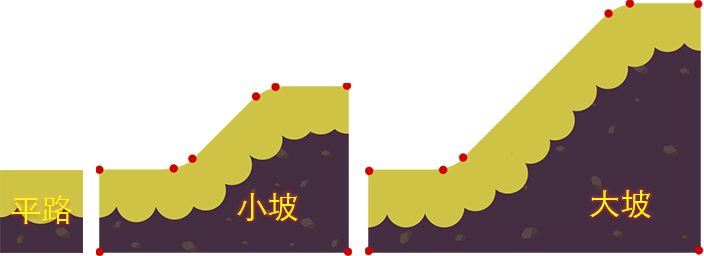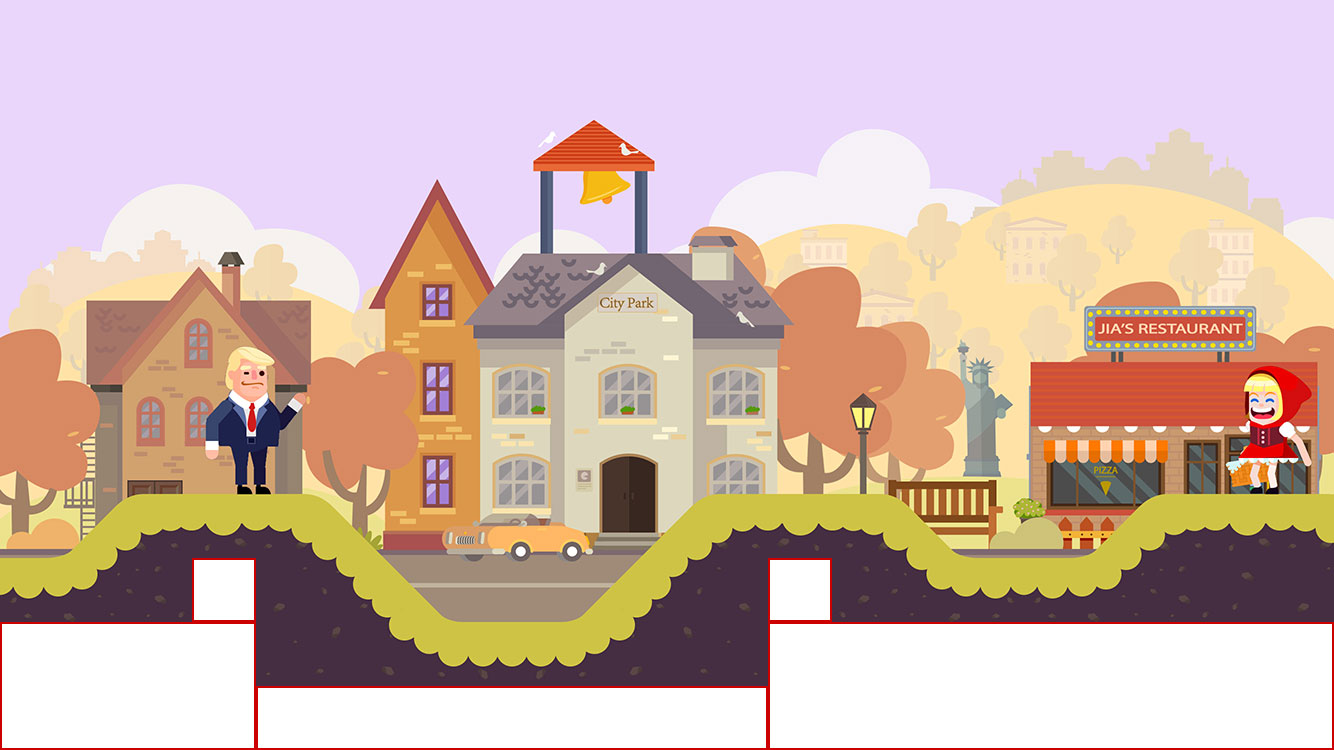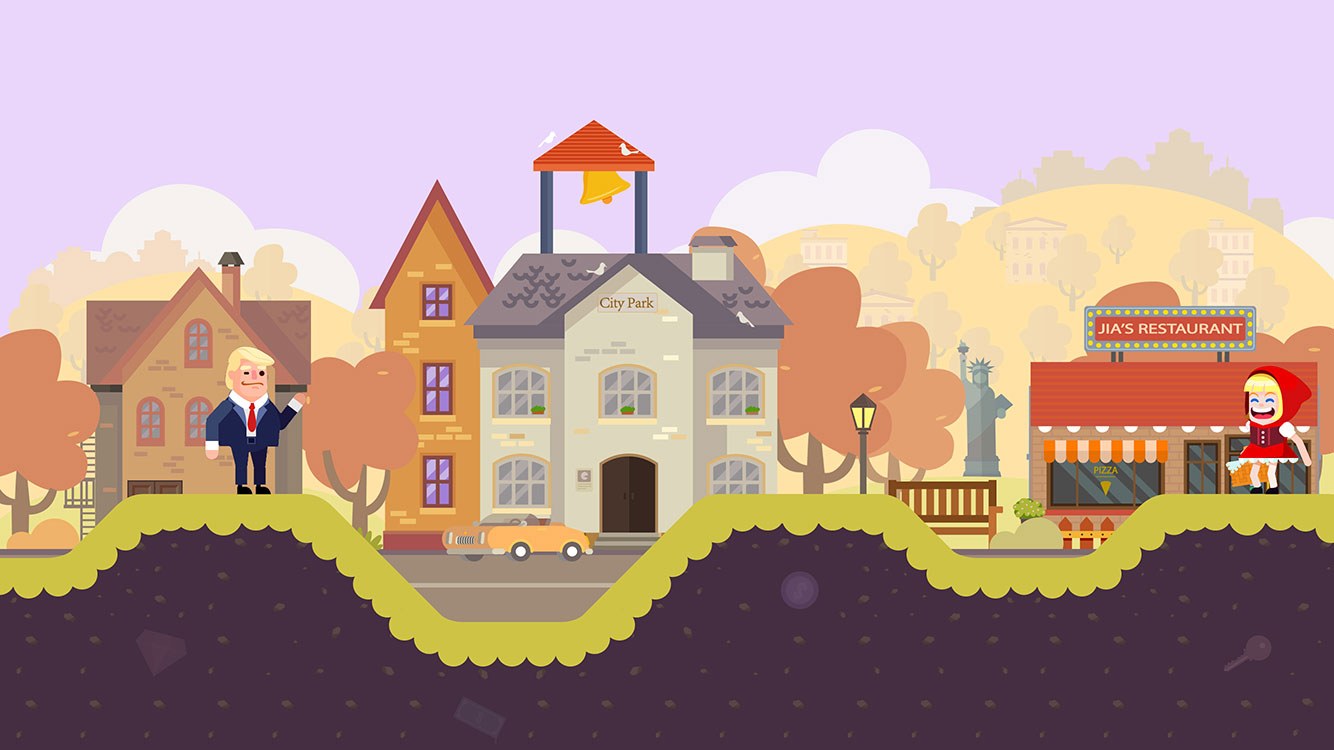• 实现容易（简单）
• 生成灵活（新奇）
• 难度可控（又好玩）

## 地形的组成

1. **平路：**type=0，size：1x1，插件：RigidBody, PhysicsBoxCollider
2. **小坡：**type=1，size：3x2，插件：RigidBody, PhysicsPolygonCollider 红色的圆点是顶点，组成多边形碰撞盒，顶点越多越平滑，当然性能也会受影响
3. **大坡：**type=2，size：4x3，插件：RigidBody, PhysicsPolygonCollider 红色的圆点是顶点，组成多边形碰撞盒，顶点越多越平滑，当然性能也会受影响</div>


## 坡路的设计思路

1. 坡的角度为45度，这样保证了物体滑落时的感觉比较自然。
2. 坡底和坡顶拐角处都包含了一块平路并且设置了2个顶点，确保物体滑落时的平滑感。
3. 坡度和坡顶都是平路可以和”平路“地形无缝连接。

## 地形数据格式

[
{"type": 0, "x": 0, "y": 0},
{"type": 0, "x": 28, "y": 0},
{"type": 0, "x": 256, "y": 0},
{"type": 1, "x": 384, "y": 0},
{"type": 0, "x": 768, "y": 128},
{"type": -1, "x": 896, "y": 0}
]


## 地形的控制

1. 战场宽度：目前是一个固定宽度8000px
2. 最大高度：地形顶部可触及高度
3. 最小高度：地形底部最低高度
4. 最大连续平路：连续平路的最大数量
5. 最小连续平路：连续平路的最小数量

## 最后附上战场地形实现示意图## gound.ts 部分代码实现

@ccclass
export default class Ground extends cc.Component {
// 纹理的 plist
atlas: cc.SpriteAtlas = null
// 纹理组件
tile: cc.Prefab = null
// 平路组件
flat: cc.Prefab = null
// 小坡组件
ramp1: cc.Prefab = null
// 大坡组件
ramp2: cc.Prefab = null

@property(cc.Integer)
sceneWidth: number = 8000
@property(cc.Integer)
maxHeight: number = 5
@property(cc.Integer)
minHeight: number = -2
@property(cc.Integer)
maxStraightParts: number = 4
@property(cc.Integer)
minStraightParts: number = 2
// 起始方向：1为朝右(正)，-1为朝左(反)
@property(cc.Integer)
direction: number = 1
// 起始高度(台阶)
@property(cc.Integer)
stairs: number = 0

// 每个体积单位的实际像素
sizeUnit = 128

// 地形使用了缓存类管理（内部使用的是cc.NodePool）
this._nodeCacheManager = new NodeCacheManager()
}

// 设置纹理主题（配合背景），之后动态更换组件的 spriteFrame
setAtlas (atlas) {
this.atlas = atlas
}

// 根据server发来的地形数据动态生成
onSyncGround (groundData: any[]) {
for (let i = 0; i < groundData.length; i++) {
// 取出每一块的数据
let prefabData = groundData[i]
// 根据地形类型取出组件并实例化到界面上
let prefab = this._getPrefab(prefabData.type)
node.getComponent(cc.Sprite).spriteFrame = this.atlas.getSpriteFrame(prefabData.type)
// 坡路可能需要翻转
if (prefab.size.h > 0) {
node.scaleX = prefabData.type > 0 ? 1 : -1
}
node.x = prefabData.x
node.y = prefabData.y

// 每铺一块地形，计算出下块地形坐标
this.startX += prefab.width * this.sizeUnit
// 如果是反向，则坡路由于锚点问题会造成偏移，所以此处修正一下
if (this.direction < 0 && prefab.size.h > 0) {
node.x = this.startX
}
// 根据当前地形坐标及大小创建它下面的装饰纹理
this.createTiles(prefab.size.w, prefab.size.h, node.x, node.y)
// 超过屏幕宽度后退出
if (this.startX > this.sceneWidth) {
break
}
}
// 地形创建完成后清除缓存
this._nodeCacheManager.clear()
}

// 平铺空白处
createTiles (w, h, x, y) {
// 在每块地形底部向下铺一块纹理（不超过minHeight）
// ...
// 随机创建其它风格样式砖块
// ...
}

// 以地形最底部的y点（即 minHeight 之下）算出最大平铺纹理区域
private _createMainTile (y: number = 0) {
let stails: number = Math.floor(y / 128)
let mainTile = this._nodeCacheManager.createNode(this.tile)
let sprite = mainTile.getComponent(cc.Sprite)
sprite.spriteFrame = this.atlas.getSpriteFrame('tile')
let height: number = (5 + stails) * this.sizeUnit
mainTile.setContentSize(cc.size(this.sceneWidth, height))
mainTile.x = 0
mainTile.y = y + this.minHeight * this.sizeUnit - height